Next: Conversion to and from Up: Representations Previous: Properties of average

# Conversion between Stream Representations

The signed binary representation is generally more appropriate than the dyadic representation for implementing most operations. This is because firstly there are a small finite number of digits, which means we can examine different combinations of digits at the start of input streams and treat them as individual cases, and also each signed binary digit occupies a fixed amount of space and cannot swell in size like the dyadic digit representation. Certain algorithms, however, are easier to define using the dyadic digit representation, either as an intermediate representation, or for both input and output.

The fact that we use two representations means that it is sometimes necessary to convert between them. We show here how this is performed for the stream representations. The (mantissa, exponent) representations are converted by performing the appropriate conversion on the mantissa and leaving the exponent unchanged.

Converting signed binary streams to dyadic streams is trivial, and is performed by generating a stream with dyadic ones, zeros, and minus ones in place of the corresponding signed binary digits.

Converting dyadic streams into signed binary representations is more complex. It is necessary to examine two digits of the dyadic input stream to decide one digit of output. The conversion function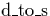is defined as follows: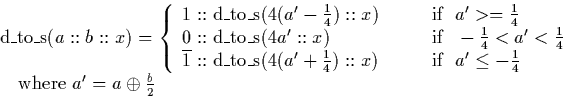The conversion function works because the value attached to the remainder of the stream x using the `cons' operation in the recursive call tois always in the range [-1,1], and as such can be represented by a single dyadic digit.

We prove of correctness as follows. The value a' represents the value of the first two digits of input. The number represented by the whole input stream is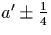because the remainder of the stream (x), which represents a value in the range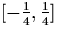. Now, if a' is greater or equal to a quarter, the entire input stream is in the range [0,1] and can be represented by the signed binary stream whose first digit is one. Similarly if a' is a value between minus a quarter and a quarter the whole stream is in the range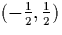and can be represented by an output stream whose first digit is zero, and if a' is a less than or equal to minus a quarter the whole stream is in the range [-1, 0] and can be represented by an output stream whose first digit is minus one. We output the appropriate digit in each case, and convert the remainder of the dyadic input stream by attaching the remainder of the output digit subtracted from a' to x and calling the conversion function recursively.Next: Conversion to and from Up: Representations Previous: Properties of average
Martin Escardo
5/11/2000